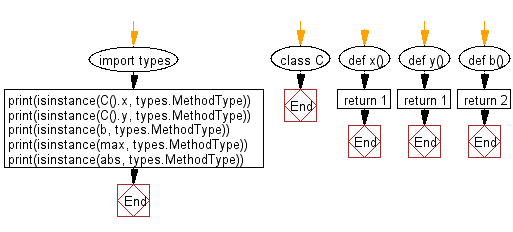﻿ Python: Check if a given value is a method of a user-defined class - w3resource# Python: Check if a given value is a method of a user-defined class

## Python module: Exercise-2 with Solution

Write a Python program to check if a given value is a method of a user-defined class. Use types.MethodType()

Sample Solution:

Python Code:

``````import types
class C:
def x():
return 1
def y():
return 1

def b():
return 2

print(isinstance(C().x, types.MethodType))
print(isinstance(C().y, types.MethodType))
print(isinstance(b, types.MethodType))
print(isinstance(max, types.MethodType))
print(isinstance(abs, types.MethodType))
```
```

Sample Output:

```True
True
False
False
False
```

Flowchart:## Visualize Python code execution:

The following tool visualize what the computer is doing step-by-step as it executes the said program:

Python Code Editor:

Have another way to solve this solution? Contribute your code (and comments) through Disqus.

What is the difficulty level of this exercise?

Test your Python skills with w3resource's quiz

﻿

## Python: Tips of the Day

For-else construct useful when searched for something and find it:

```# For example assume that I need to search through a list and process each item until a flag item is found and
# then stop processing. If the flag item is missing then an exception needs to be raised.

for i in mylist:
if i == theflag:
break
process(i)
else:
raise ValueError("List argument missing terminal flag.")
```